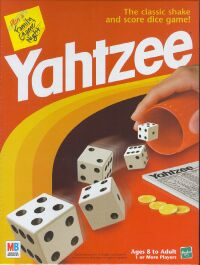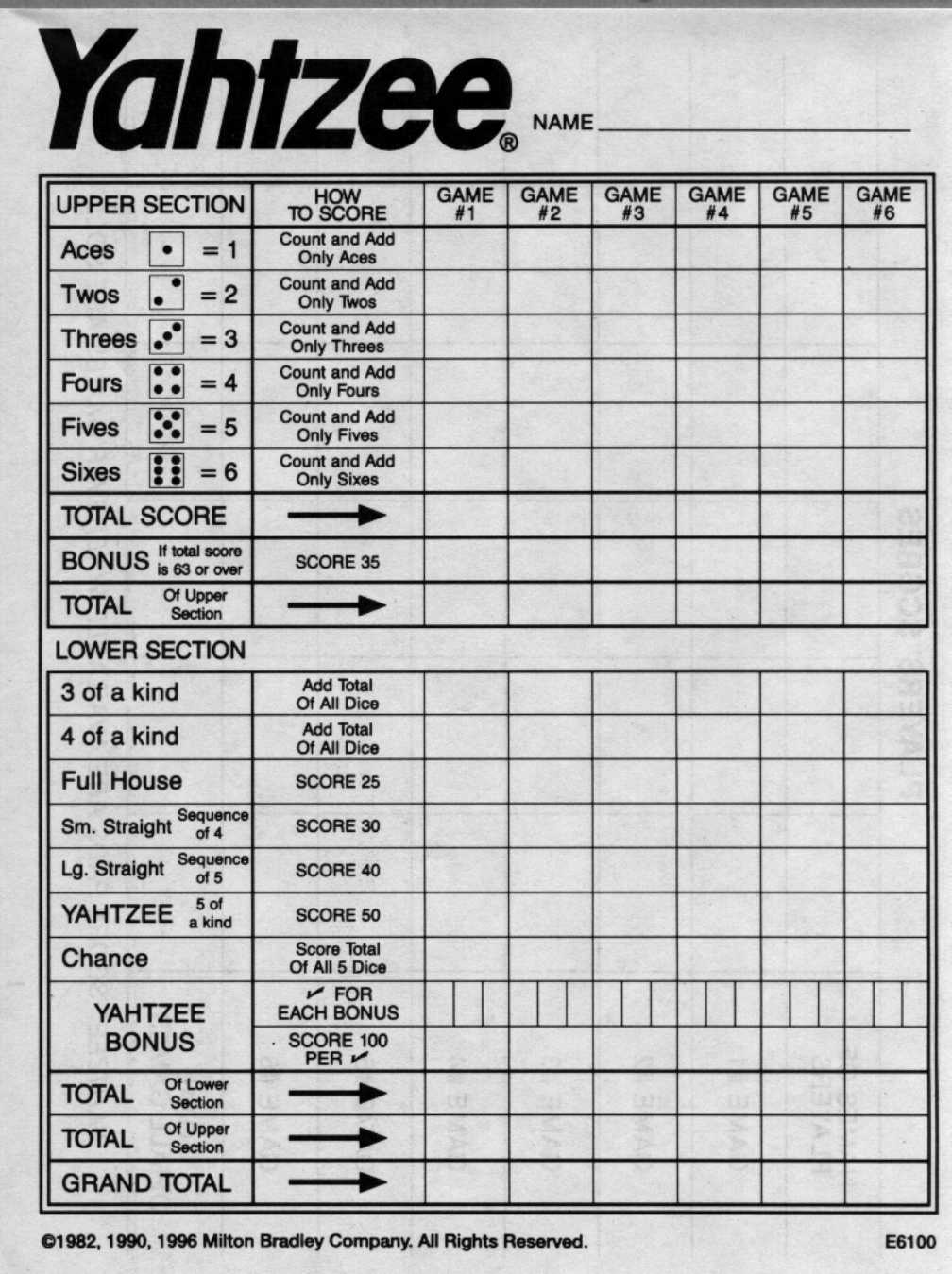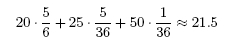# YahtzeeYahtzee is a dice game based on Poker. The object of the game is to roll certain combinations of numbers with five dice. At each turn you throw dice trying to get a good combination of numbers; different combinations give different scores. While luck plays a big role in Yahtzee, strategy makes a significant difference. The reason for this is that you score each combination just once, and the number of different combinations in which you can score is equal to the number of turns in the game. This means that you have to make wise choices about when to score in each combination and you have to be careful about what combinations you seek at each turn.

## Rules and Scoring

The game takes thirteen turns. Each turn consists of up to three separate rolls of the dice. On the first roll you roll all five of the dice. After the first and second roll, you can hold any subset of the five dice you want (including none of the dice or all of the dice) and roll the rest trying to get a good combination. After the three rolls (or after the first or second roll if you choose to stop) you must find a place among the thirteen boxes in the scorecard to put your score. The score you get depends on the box that you choose and the combination that you have rolled. After a box is used, you can't use it again, so you have to choose wisely. This means that, in general, you don't have to choose the box that gives you the highest score for the combination you have rolled, since it may be advantageous to save that box for an even better roll later in the game. In fact, there are many situations it which it makes sense to put a 0 in a "bad" box instead of a low score in another "good" box because doing so would block the good box for future turns.

The Yahtzee scorecard contains two sections: the upper section and the lower section.#### The Upper Section

In the upper section, there are six boxes, each corresponding to one of the six face values of the dice. For these boxes, you enter the sum of the dice with the corresponding face value (and ignore all other dice). For example, if you roll 5 3 3 5 3 you may enter a score of 9 in the third spot or a score of 10 in the fifth spot.

Bonus: At the end of the game, you get a bonus of 35 points if the total number of points you scored in the upper section is 63 or higher. The seemingly random number 63 corresponds to having scored a combination with three dice of the corresponding face value in each of the upper section boxes (though any upper section total of 63 or greater is rewarded with the bonus).

#### The Lower Section

Here you can score for various combinations.

• 3 of a kind: Score in this box if the dice include 3 or more of the same number. The score is the total of all five dice (unlike the upper section, where only the dice of one number are summed).
• 4 of a kind: Score in this box if the dice include 4 or more of the same number. The score is the total of all five dice.
• Full House: Score in this box if the dice show three of one number and two of another (for example, 2 6 6 2 2). The score is 25 points.
• Small Straight: Score in this box if the dice show any sequence of 4 numbers (for example 3 2 4 4 5). The score is 30 points.
• Large Straight: Score in this box if the dice show any sequence of 5 numbers (for example 5 2 4 6 3). The score is 40 points.
• Chance: Score in this box with any combination. The score is the total of the five dice.
• Yahtzee: Score in this box if the dice show five of the same number. The score is 50 points.
• Yahtzee Bonus: If you roll a second Yahtzee and the Yahtzee box is already filled with 50 points, you get a 100 points bonus. If you roll a Yahtzee and your Yahtzee box is filled with a zero, you do not receive this bonus. In either case, you must fill one of the other boxes on your scorecard using the "joker rules" as follows: if possible, you must fill the upper section box corresponding to the number you rolled. If that box is already filled, you must fill in any open lower section box. For the 3 of a kind, 4 of a kind, and chance boxes, this means scoring the total of all 5 dice. For the full house, small straight, and large straight boxes, this means scoring 25, 30, or 40 points respectively (even though you did not actually roll the corresponding combination). If the entire lower section is filled you must enter a zero in any open box in the upper section.

## Strategy

Now we are going to review a little bit of strategy for playing Yahtzee.

#### Basic Tips

The following are generally regarded as the basic things to keep in mind while playing Yahtzee.

• You should generally aim for the 35 points bonus by filling the upper section with high scores (so as to get at least 63 points) near the beginning of the game.
• If you roll a combination for which your available boxes give a low score, fill in a zero in the 1's box (or even the 2's or 3's), since high scores in the other boxes may compensate for this.
• Early in the game you should use the chance box only as a fall-back for when you are trying for a high point combination and fail. You want to keep the chance box available for a situation where your options are very limited.
• If you have a bad roll of the dice near the end of the game, you may enter a zero in the Yahtzee box or one of the other difficult combinations.
• If you roll a pair of 1's or 2's early in the game, go for a full house, straight, or one of the other high numbers you rolled. Keeping the 1's or 2's to seek a 3 or 4 of a kind will not get you many points. Plus, it is a good idea to keep the 1's and 2's boxes available for later in the game to have the possibility of putting a zero there. As an example, if on your first turn your initial roll is 1 1 2 5 6, it turns out that keeping only the 5 is the choice that maximizes your expected score for the game.
• If you roll four of a kind with 4's, 5's, or 6's, take the points in the upper section if they are available, not in the 4 of a kind box. This will help you get the 35 points bonus at the end of the game.
• You should always try for a Yahtzee early in the game. If you roll a 3 or 4 of a kind on your first or second roll and you have the corresponding box in the upper section open, you should definitely go for the Yahtzee. Plus, if you saved your chance, 1's, or 2's boxes, you can score a zero there if you fail to get the Yahtzee.

Any kind of advanced strategy for Yahtzee has to rely on calculating or estimating the expected final score you will get with respect to your current position in the game and the different possibilities you have for scoring. This is a very difficult task, since in general it involves too many calculations. As with most board games, the best way to actually apply this principle is by playing a lot and developing some intuition as to what the best choices are. In general, you should be able to discard many possibilities just by looking at them, and then choose among the remaining ones in the best way you can.

To get a grasp of the kind of calculations you would need to do in order to determine the best move in Yahtzee, we will consider the following simplified example.

Suppose that you are playing a "1-turn Yahtzee" game (all the boxes are empty and you are tying to maximize your score for a single turn), and your second roll leaves you with 3 3 3 1 1. Which (if any) of the dice should you keep for the last roll?

To solve this, observe first that since this is the 1-turn version of Yahtzee, all boxes are available and your only goal is to get the highest possible score in this turn. It should be clear that the only sensible choices are keeping everything (scoring 25 points for a full house) or re-rolling both 1's (trying for a Yahtzee). What is the best choice?

If you keep everything, the best score you can get is scoring the full house box, and you get 25 points. If you re-roll both 1's, three things may happen:

1. You get two different numbers. This will happen with probability 5/6. In this case, your best score corresponds to either the 3 or 4 of a kind box, where you add up the five numbers. In this case, the maximum score you can get is 20 (if you rolled a 5 and a 6).
2. You get two equal numbers, different from 3. This happens with probability (5/6) * (1/6) = 5/36. In this case you rolled a full house, and it is the best box to score in this case, since any other possibility scores at most 3 + 3 + 3 + 6 + 6 = 21 points. Thus, you score 25 points.
3. You get two 3's. This will happen with probability 1/36, and you score 50 points for a Yahtzee.

With all this, we can say that the average score you get if you re-roll the 1's is less thanThus, we see that your average score when re-rolling is less than 25, so you should keep all the dice and score a full house. As you observe, even in this very simplified situation, the calculations get impossible to carry out during a game.

You can find more information on Yahtzee's rules, strategies and history on the Internet. For instance, you can try Wikipedia.

You can find an optimal Yahtzee player and proficiency test at here.

Problems

Some of the problems are very easy, but some are more involved, especially the last two. You may want to go over the review of basic probability before trying them.

1. If you roll a die three times, what is the probability that you will get at least one six?

2. Suppose that on the first roll you get 5 5 4 3 2. If you pick up one of the fives and try for a large straight, what is the probability you will succeed in the two rolls that remain?

3. Suppose that on the first roll you get 6 5 5 3 2. If you pick up one of the fives and try for a large straight, what is the probability you will succeed in the two rolls that remain? Is it the same as in the previous problem?

4. In the top six rows of the score card you want to score at least three of a given number (especially for the high numbers like 5 and 6). Suppose you roll 6, 6, 5, 2, 1 the first time, and re-roll all non 6's on the next two tries. What is the probability you will end up with at least three 6's? Exactly four 6's? A Yahtzee (five 6's)?

5. Suppose on the first roll you get 5, 4, 4, 3, 1. To try to get a large straight you pick up a 4 and the 1 and then roll again. If you get a 6 or 2 you keep it, and you re-roll the others. Of course if you happen to get a 2 and 1 on the second roll you stop and are happy. What is the probability you will end up with a large straight? What is the probability you will end up with a small straight?

Suppose instead you keep 5, 4, 3, 1, and re-roll 1 die. If you get a 2 you are happy. If you get a 6 you re-roll the 1. Otherwise you take a second chance at rolling the 2. What is the probability you will end up with a large straight? a small straight?

6. Suppose you are playing a game where you can roll a single die one or two times. Your objective is to get a high number. After the first roll you can choose whether to keep the number you got or re-roll. Then the best strategy is to keep a 4, 5, or 6, while re-rolling a 1, 2, or 3 (try to convince yourself of this). Now suppose we play the same game but with the option of rolling the single die one, two, or three times. As before, after the second roll you should keep a 4, 5, or 6, and re-roll a 1, 2, or 3. Can you come up with a strategy on what to do after the first roll such that the expected (or average) score you get in the three rolls game is better than the expected score in the two rolls game with the strategy we proposed? What is the expected score when playing with your proposed strategy? These types of calculations are useful in the actual Yahtzee game, for example when you are trying to score well in the chance box.

7. It is the last roll of the game and you need a Yahtzee to win. After the first roll you keep the dice of the most common number you rolled and then re-roll twice to see if you can end up with all of that number. (We ignore the possibility that you change the number for which you are looking after the first roll, e.g., you only had one 5 after the first roll and then rolled two 4's.) In the case of a tie on the first roll, choose one of the numbers that appears the maximal number of times and keep only the dice with that number. What is the probability you end up with a Yahtzee? This problem is rather difficult, so don't expect to get a fast answer. Here is a hint: first compute the probability of getting 2, 3, 4, or 5 of a kind, or all different numbers in the first roll; then try to compute the probability of passing from having i of a kind in a roll to having j on the next roll and try to use these "transition probabilities".

8. In reality, if you are going for a Yahtzee and you keep two 4's after you first roll but roll three 5's on your second roll, you will try for a Yahtzee in 5's on your last roll. Repeat the previous problem, but now incorporating the fact that after your second roll you may change the number that you are seeking. Once again, if there is a tie, choose one number to keep. If you get all different numbers on your first roll, re-roll all of the dice.